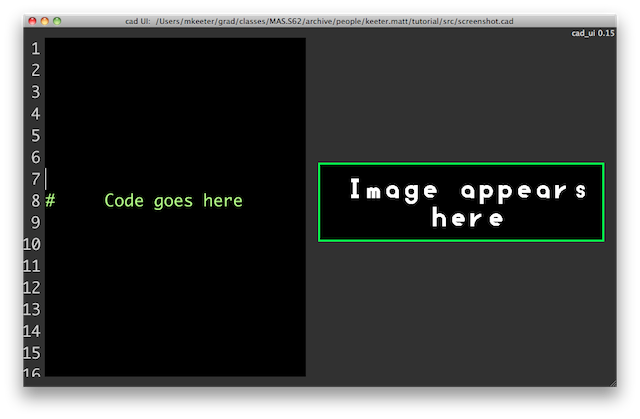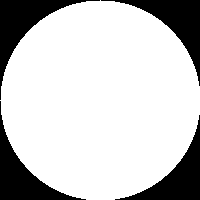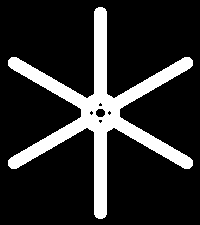HTMSTM(a)A / People / Matt Keeter / Tutorials

# Designing with Code

## Prerequisites

To play along at home, you will need the `cad_ui` application.

It is available as a bundled Mac application or as source. Note that the source archive includes the rest of the fab modules. These are wonderful tools but will not be covered in this limited tutorial.

If you're building from source, the software uses cmake to automate the build process. Details about dependancies are in the archive's `INSTALL` file. Good luck!

## What is a `.cad` file?

A `.cad` file is a specialized Python script used to define an object. The only requirement is that the file defines the following variables (all stored within a structure named `cad`):

• `cad.xmin`, `cad.xmax`, `cad.ymin`, `cad.ymax` define the region of interest.
• `cad.function` defines the shape of the object.

The following optional variables may also be defined:

• `cad.zmin` and `cad.zmax` define the region for three-dimensional objects.
• `cad.mm_per_unit` defines the number of mm per cad unit (default is 1)
• `cad.type` can be either "Boolean" or "RGB" (default is "Boolean")

## The Interface

The `cad_ui` tool has a two-pane interface.The file is written on the left, and its interpretation into an image appears on the right.

The border of the image indicates status, which is also printed below the image when trouble occurs.

• Green indicates that the image is valid and up-to-date.
• Orange means the image is being updated.
• Red indicates that something has failed.

To see more details on an error, enable "Show errors" option in the "View" menu. Below is a failed attempt to create a circle. Note that the line with the error is marked with a red arrow in the left margin.## Simple Example

The design tool comes with two libraries: `cad_shapes` and `cad_text`.

Let's use the `circle` function from `cad_shapes` to make a simple example:

 `````` from cad_shapes import * cad.xmin = -1 cad.xmax = 1 cad.ymin = -1 cad.ymax = 1 cad.function = circle(0, 0, 1) ``````For reference, this is what the example looks like in `cad_ui`.

## Logical Operations

The standard library includes `add`, `subtract`, `intersect`, and `invert` operations. Let's cut a rectangle out of our previous example.

 `````` from cad_shapes import * cad.xmin = -1 cad.xmax = 1 cad.ymin = -1 cad.ymax = 1 outer = circle(0, 0, 1) inner = rectangle(-0.5, 0.5, -0.5, 0.5) cad.function = subtract(outer, inner) ``````Note that if you start typing a function from the standard library, the GUI will give you a syntax hint in the botton left corner.## Programmable Design

Because the `.cad` file is a Python script, it is easy to create complex objects than lend themselves to programmatic expression. The example below shows a cutout being rotated about the origin and repeatedly removed from the base part.

 `````` from cad_shapes import * cad.xmin = -1 cad.xmax = 1 cad.ymin = -1 cad.ymax = 1 part = circle(0, 0, 1) cutout = rectangle(-0.2, 0.2, -0.2, 0.2) cutout = rotate(cutout, 45) cutout = move(cutout, 0, 0.6) for i in range(6): part = subtract(part, rotate(cutout, i * 60)) cad.function = part ``````## Practical Example

Below is an actual part designed as a `.cad` script. The part is a stand for a brushless DC motor, used to keep it steady for benchtop testing.

 `````` from cad_shapes import * # All measurements are in inches hole_radius = 0.125 / 2 hole_r_a = 0.65 / 2 hole_r_b = 0.745 / 2 motor_r = 0.345 / 2 stand_r = 4.0 num_legs = 6 # Create a multi-legged base to stay stable leg = rectangle(-0.25, 0.25, 0, stand_r) leg = add(leg, circle(0, stand_r, 0.25)) base = circle(0, 0, 0.8) for i in range(0, num_legs): base = add(base, rotate(leg, 360 * i / num_legs)) # Create cutouts for the motor shaft and screws holes = circle(0, 0, motor_r) holes = add(holes, circle(0, hole_r_a, hole_radius)) holes = add(holes, circle(0, -hole_r_a, hole_radius)) holes = add(holes, circle( hole_r_b, 0, hole_radius)) holes = add(holes, circle(-hole_r_b, 0, hole_radius)) # Render boundaries cad.xmin = -4 cad.xmax = 4 cad.ymin = -4.5 cad.ymax = 4.5 cad.mm_per_unit = 25.4 # inch units cad.type = "Boolean" # Boolean or RGB cad.function = subtract(base, holes) ``````## Next Tutorial

Now that we've covered the basics, the next tutorial goes behind the curtain to explain how objects are represented and manipulated.